# Solution of Triangle (Sine Rule and Cosine Rule)

1.Introduction :

In any triangle three sides and angle of three sides are called element of a triangle.When three elements are given and processing of other element called Solution of Triangle.

In any triangle ABC :

• A+B+C = 180 degree
• a+b>c ,b+c>a, a+c>b
• a,b,c>0

SINE RULE:

In any triangle ABC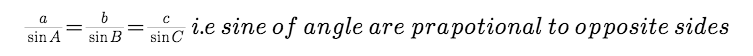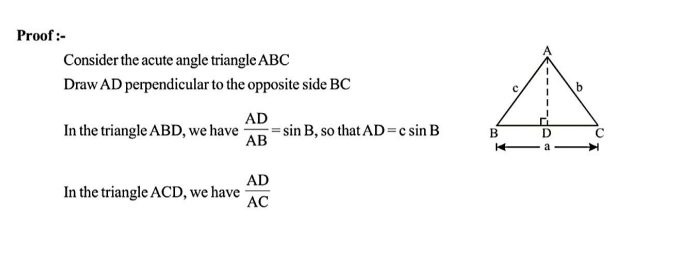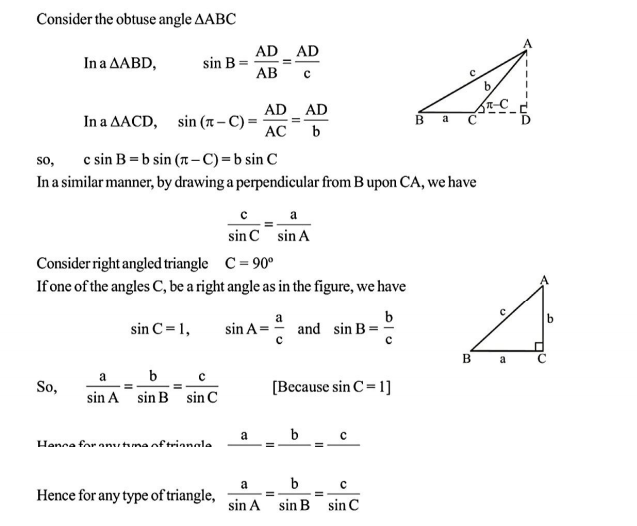Cosine Rule: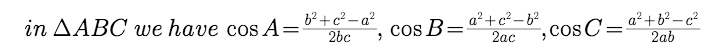where a,b,c are sides and A,B,C are angle of triangle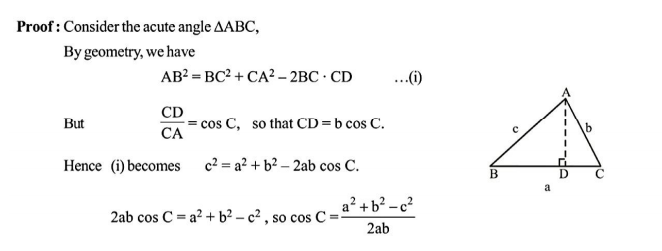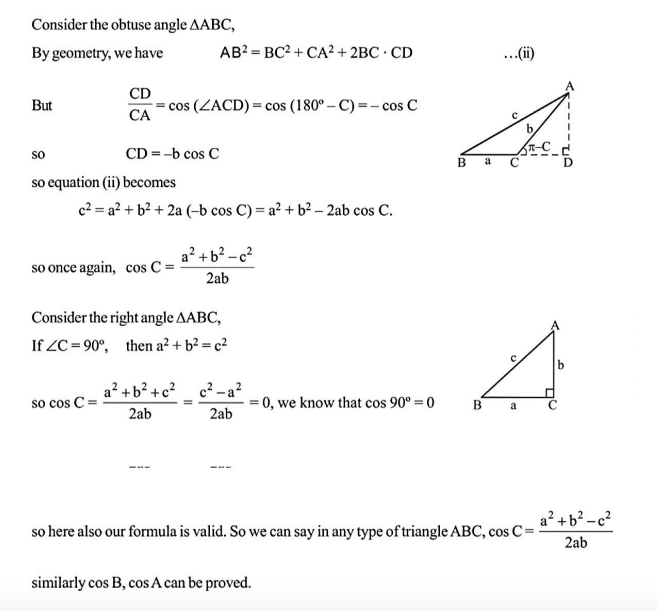Post By : Dipanker Mehata 14 Sep, 2018 4539 views Maths JEE MAIN IIT